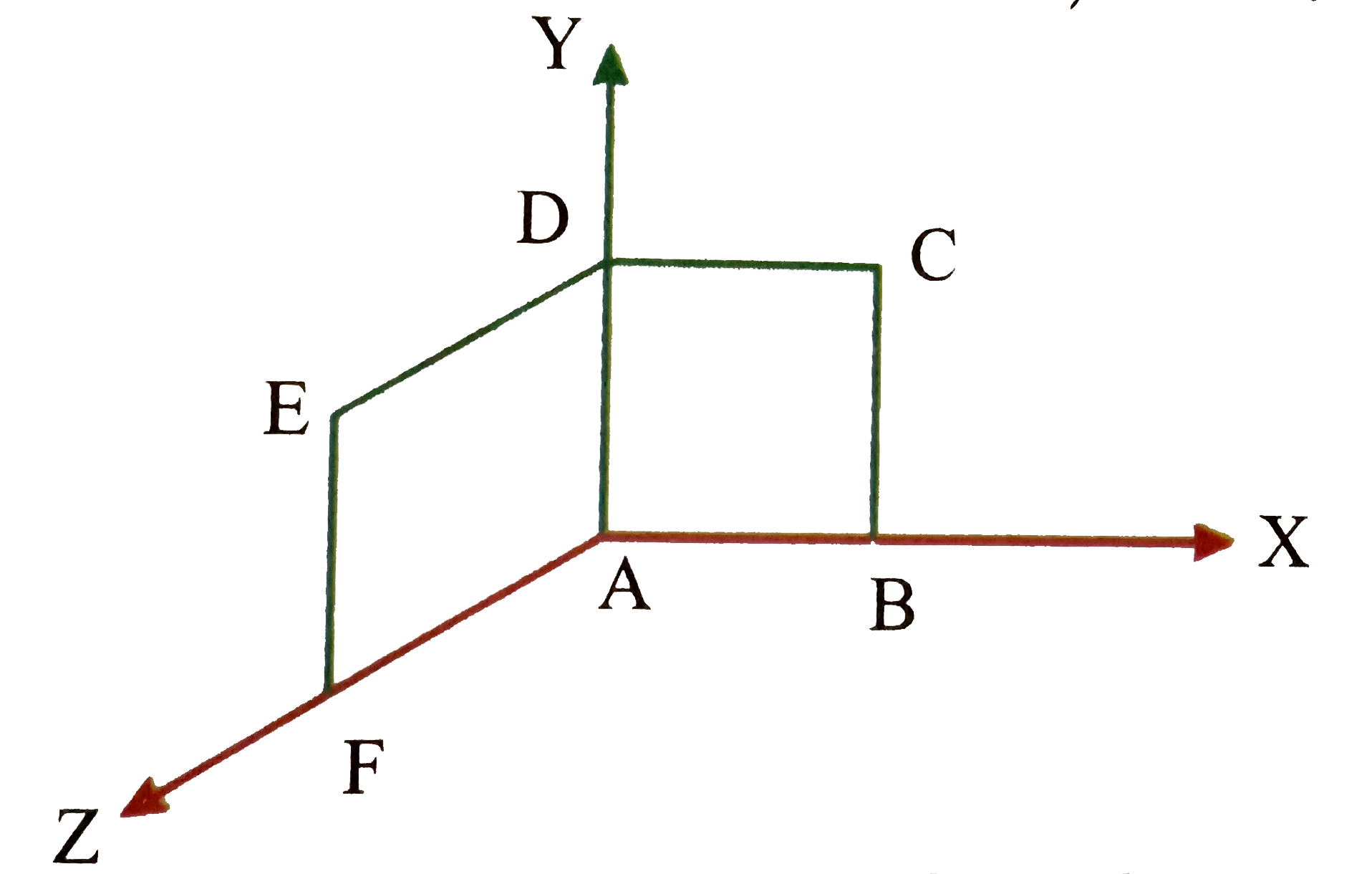Doubtnut App - Get 50 Lakh+ video solutions better than GoogleInstall Now

# A loop made of straight edegs has six corners at A(0,0,0),B(L,O,0)C(L,L,0),D(0,L,0)E(0,L,L) and F(0,0,L). Where L is in meter. A magnetic field B=B0(ˆi+ˆk)T is present in the region. The flux passing through the loop ABCDEFA (in that order) is

A

B0L2Wb

B

2B0L2Wb.

C

2B0L2Wb.

D

4B0L2Wb.

Text Solution
Verified by Experts

## Also the magnetic flux linked with uniform surface of area A in uniform magnetci field given by ϕ=B.A A=A1+A2=(L2ˆk+L2ˆi) and B=B0(ˆi+ˆk)T Now ϕ=B.A=B0(ˆi+ˆk).(L2ˆk+L2ˆi)=2B0L2Wb